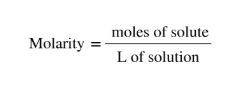# Problem: What volume of 0.0105 M HBr solution is required to titrate 125 mL of a 0.0100 M Ca(OH)2 solution?Ca(OH)2(aq) + 2HBr(aq) ⟶ CaBr2(aq) + 2H2O(l)

###### FREE Expert Solution
• We will be using the V and molarity of Ca(OH)2 to find moles of Ca(OH)2• Stoichiometric ratio from the balanced equation will be used to find the moles of HBr from moles of Ca(OH)2

82% (47 ratings)###### Problem Details

What volume of 0.0105 M HBr solution is required to titrate 125 mL of a 0.0100 M Ca(OH)2 solution?

Ca(OH)2(aq) + 2HBr(aq) ⟶ CaBr2(aq) + 2H2O(l)